Start typing, then use the up and down arrows to select an option from the list.# Combustion Analysis Example 1

Jules Bruno
347views
1
1
will now take a look at the combustion of carbon compounds. Here in this example, problem in states, a 0.2500 g sample contains carbon, hydrogen and oxygen and undergoes complete combustion to produce 0.3664 g of carbon dioxide and 0.1500 g of water. What is the empirical formula of the compound? All right, so this is typical of any combustion analysis type of question, and usually we'll have the production of sealed to in water. Now, these are the steps we're gonna have to undergo in order to determine the empirical formula off our compound. Now, Step One says that we have to convert the grams of carbon dioxide, which is co two into just grams of carbon now within C 02 We have a C in there, so we're just trying to figure out how many grams of C R within it. We're going to start out with 0.3664 g of co two. The first step is to get rid of these grams of co two. So grant of seal to go on top and on the bottom and on top, we have one mole of CO. Two now. At this point, we need to determine the molar mass of carbon dioxide. Carbon dioxide is composed of one carbon and two oxygen. Their atomic masses from the periodic table are 12.1 g and 16 g, respectively. Multiply everything out and then add those numbers together and we'll have the mass of carbon dioxide as being 44 g. So we're gonna be dealing a lot with carbon dioxide when it comes to combustion analysis type of questions. So it's pretty important to remember the Mass of CO two comes from one carbon into oxygen's. At this point, the grams cancel out and we have moles of Co. Two. We're going to stay here for every one mole of co two. We have exactly one mole within its formula. If you look at co two, there's one carbon for two oxygen's. So for every one mole of the whole thing, we see that there's only one card, one mole of carbon. At this point, moles of carbon dioxide cancel out and now we have moles of C. Remember, we don't want to just stop it. Moles of See, we want to stop at grams of C. We're going to stay here for every one mole of carbon. It's mass again. According to the periodic table is 12. with moles of carbon. Cancel out. We'll have now the grams of carbon, which comes out to be 0. g of C first step to we're gonna do the same thing with water, but here, we're gonna find the grams off hydrogen. Now, the reason that we're finding grams of carbon and hydrogen first is because they're located in only one place. See is only located in CO two and H is only located in water. It be hard for us to determine the amount of grams of oxygen because oxygen is present within both compounds. Okay, so again we find carbon and H first because they're located in only one of each of those compounds we have here 1500 g of water. We're gonna convert grams of water into moles of water, so grams of water go on the bottom. Now we need to determine the Moller Mass of water. Water has in it to hydrogen and one oxygen their masses, according to the periodic table are 1.8 g and 16 g. So that is 2016 g and 16 g. So that comes out to 18 06 g. So here grams of water, cancel out. Now I have moles of water. At this point, we're gonna say for every one mole of water, we can see that the formula contains two hydrogen. So for every one mole of water, we have two moles of H. So moles of water cancel out now have moles of H For every one mole of H, it's mass is 1.8 g h. So now we're gonna get 0. g of h. So we found out how many grams of h grams of carbon within which exists within our sample. So that takes us to step three in Step three, We say, if necessary, subtract the grams of step one and two in the grams of the sample to determine the third element in this case, we're told that our sample has in it carbon, hydrogen and oxygen, and we're told that the sample ways 0.2500. So it has carbon, hydrogen and oxygen in it. So subtract out the grams of carbon, plus the grams of hydrogen, and what will be left would have to be my grams of oxygen. All right, so when we do that, we're gonna get 4 g of oxygen 40.1332 to 5 g oxygen. Now, this is important, because now that we know the grams of each of the elements, the steps kind of become familiar to us, because at this point, we could determine the empirical formula. So here we're going to step four, we're gonna convert all the masses that we've just isolated from our three elements into moles. So take each one of those sample amounts that you just got each one of these element amounts that you just got and convert them all into molds. So remember, we divide them all by their atomic masses, and we'll have the moles of each of them so we can see that this process is not a quick process. It does require a good deal of steps, so make sure you follow each of these steps thoroughly to get your final answer. And then we have 0.1 332 to 5 g of oxygen. And that's one mold. 0 16 g of Oh, so when we do that, we're gonna get for the moles. For each we're gonna get 0.833 moles of C We're gonna get 0167 moles of H We're gonna get 833 moles of O That leads us into step five. We're going to divide each bowl, answer by the smallest small value in order to obtain whole numbers for each element. The smallest mall answer that we got was 0. So each of them gets divided by that. So when we do that, we're going to get one C to H. One of now. This will give us our empirical formula of C H. 20 Now let's say we didn't get whole numbers. Then we go into step six and Step six says if you get a value meaning these numbers here and they had a 0.1 or nine, then you can round to the nearest whole number. So if you got 1.1, you go round down to one. If you've got 2.9, you could round up to three. Now, if you can't round that, we'd multiply them all by an integer a number in order to create whole numbers. In this case, we didn't have to do that. Now, in this case, based on the steps that we've taken, the empirical formula for our compound would be C H 20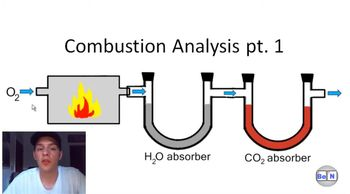11:51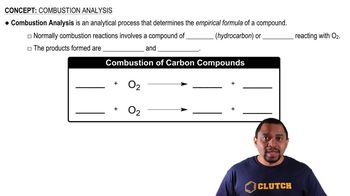02:09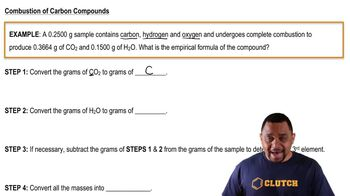08:13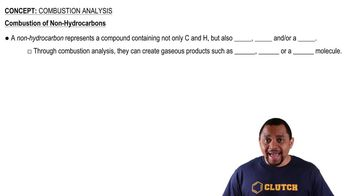01:29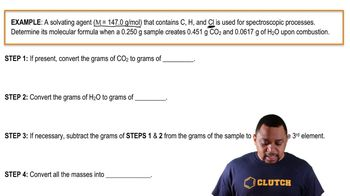08:32